OpenWindows User's Guide

# Chapter 8 Calculator

The DeskSet Calculator is a scientific and financial calculator designed to perform a variety of mathematical functions. It operates in much the same way as many hand-held calculators. There are financial, logical, and scientific modes. You can store numbers in ten different memory registers and retrieve and replace them easily. In addition, you can store up to 10 of your own functions and constants in menus.

The Calculator displays numbers in fixed-point notation and decimal base, but you can change it to display numbers in engineering or scientific notation, and in binary, octal, or hexadecimal base.

This chapter describes how to use the Calculator. You can use Calculator with a mouse, as you do other OpenWindows applications, or without a mouse, as you would a physical hand-held calculator. With the mouse, you click SELECT on the Calculator buttons; otherwise, you use the keyboard.

To open the Calculator application, choose Workspace -> Programs Calculator.

# Calculator Icon and Base Window

The Calculator icon and base window are shown in Figure 8-1. The base window has six rows of eight buttons. Click SELECT on a button to activate it.

##### Figure 8-1 Calculator Icon and Base Window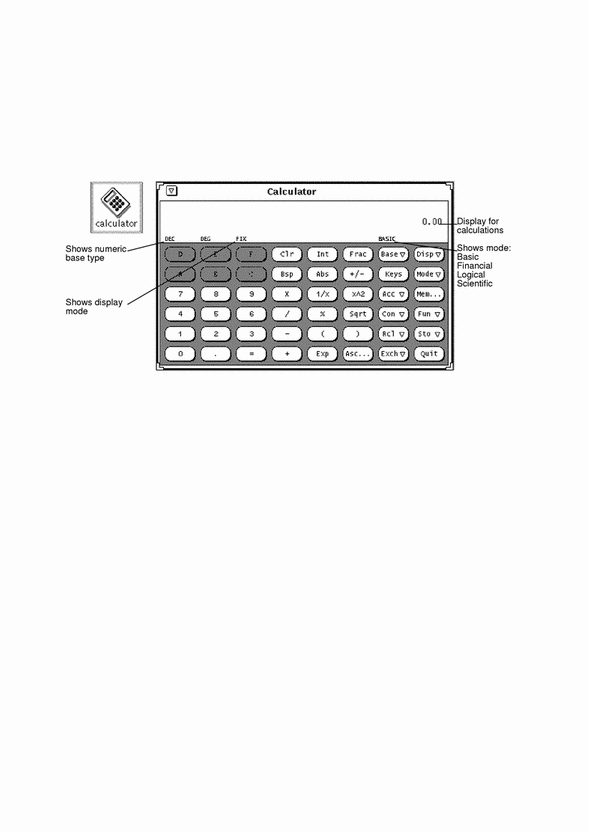The Calculator base window is resizable by using the resize corners (it might take a few seconds to redisplay when it's resized).

# Modes of Operation

The Calculator has various modes of operation. The area below the window header displays the current number, followed by a mode line that displays the current mode settings.

## Numeric Bases

You can set the numeric bases to Binary, Octal, Decimal, or Hexadecimal using the Base key pop-up menu, as shown in Figure 8-2. Press MENU on the Base key to display the menu.

##### Figure 8-2 Numeric Base Pop-up Menu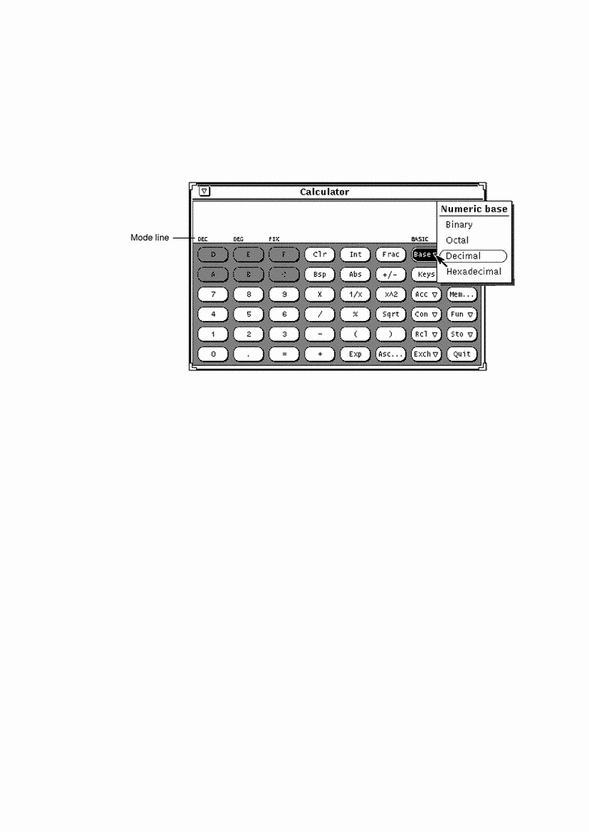The current base setting is shown as the first item in the mode line. In Figure 8-2, "DEC" indicates Decimal base.

Up to 40 digits can be entered for each number, regardless of the base. If you need to enter a larger or smaller number, use the Exp key as discussed under "Number Manipulation Functions". The numeric keypad changes to display only those numbers appropriate to the current base mode. Inappropriate numbers are dimmed out. For example, in Figure 8-2 the hexadecimal letter keys (A-F) are dimmed. The numeric base in that figure is decimal, so the hexadecimal letters are not valid or accessible.

• Choosing Binary displays the digits 0 and 1 on the numeric keypad.

• Choosing Octal displays the digits 0 through 7.

• Choosing Decimal displays the digits 0 through 9.

• Choosing Hexadecimal displays the digits 0 through 9 and also activates the alphabetic keys at the top of the numeric keypad.

Figure 8-3 shows the keypads for both Binary and Hexadecimal numeric bases.

##### Figure 8-3 The Binary Keypad and Hexadecimal Keypad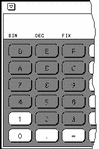## Display Notation

You can choose to display Calculator results in Fixed point, Engineering, or Scientific notation by using the Disp (Display) key menu shown in Figure 8-4. Press MENU on the Disp key to display the menu.

##### Figure 8-4 Display Type Pop-up Menu for Display Notation Mode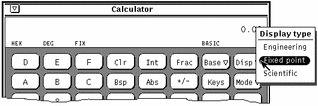The current display notation is displayed as the third item in the mode line. In Figure 8-4, the mode line shows FIX, indicating Fixed Point notation. When the current display notation is Engineering, ENG appears on the mode line. When the current notation is Scientific, SCI is displayed.

When the current notation is Fixed Point, the Calculator will revert to Scientific notation if a number is larger than the display will allow.

## Major Calculator Modes

There are four major Calculator modes that determine the available functions:

• Basic

• Financial

• Logical

• Scientific

You can choose these four modes using the Mode pop-up menu shown in Figure 8-5. Press MENU on the Mode key to display the menu. The default mode is Basic mode, which uses the standard keys on the Calculator window. The other three modes have extra buttons that appear in a pop-up window. For information on these modes, see "Financial Functions", "Logical Functions", and "Scientific Functions".

##### Figure 8-5 Mode Pop-up Menu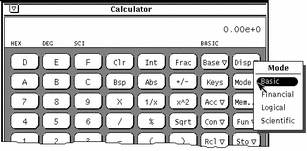##### Figure 8-6 The Mode Menu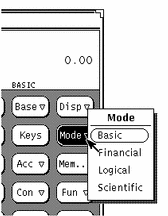The current major mode is displayed on the mode line underneath and slightly to the left of the number display. In Figure 8-5, BASIC is listed as the current mode.

## Number Manipulation Functions

The Calculator has 12 number manipulation functions, as shown in Figure 8-7.

##### Figure 8-7 Number Manipulation Functions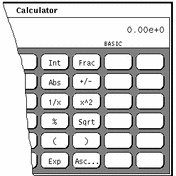• Int returns the integer portion of the currently displayed value.

• Frac returns the fractional portion of the currently displayed value.

• Abs returns the absolute value of the currently displayed value.

• +/- changes the arithmetic sign of the currently displayed value or of an exponent being entered with the Exp key.

• 1/x returns the value of 1 divided by the currently displayed value.

• x^2 returns the square of the currently displayed value.

• % calculates the percentage of the currently displayed value specified by the next value entered. For example, to find 75% of 40, enter 40, click SELECT on %, enter 75, then click SELECT on =. The Calculator returns the result, 30.

• Sqrt returns the square root of the currently displayed value.

• Parentheses give a calculation arithmetic precedence. All calculations within parentheses are calculated first. Parentheses can be nested. The result of an operation containing parentheses is not performed until the last parenthesis is matched.

• Exp starts exponential input. Any number entered after you choose Exp is taken to be the exponent portion of this number. The numbers entered before the Exp key are taken to be the mantissa. If no numerical input has occurred when the Exp key is entered, a mantissa of 1.0 is assumed. You can use the +/- key after entering the exponent to change its arithmetic sign.

• Asc displays the ASCII value of a character in the appropriate numeric base. Clicking on the Asc key displays a small pop-up window. Type the character that you want an ASCII equivalent for in the Character text field of the Get ASCII window, and then click SELECT on the ASCII button. The ASCII equivalent becomes the current value in the Calculator base window.

# Miscellaneous Functions

The Calculator has five miscellaneous functions, shown in Figure 8-8.

##### Figure 8-8 Miscellaneous Functions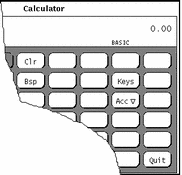• Clear (Clr) clears the current value from the display.

• Backspace (Bsp) deletes the rightmost character of the current display and recalculates its value.

• Keys changes the display of the Calculator keys to show the keyboard equivalents of each Calculator key for mouseless operation of the Calculator.

• See the section "Calculator Function Keys", or the calctool manual page, for more information about the Calculator keyboard mapping.

• Accuracy (Acc) chooses the number of digits of precision used in the calculator display. This key has a pop-up menu associated with it, which lets you specify 0 through 9 radix places. The default value is 2 radix places.

• Quit quits the Calculator.

# Memory Registers

The Calculator has 10 memory registers that you can access using the keys shown in Figure 8-9.

##### Figure 8-9 Memory Register Keys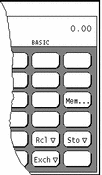• Memory (Mem) displays a pop-up window showing the values of the 10 memory registers in the current base to the current accuracy.

• Store (Sto) stores the current value in the memory register that you choose from the Store pop-up menu.

• Recall (Rcl) retrieves a value from the memory register that you choose from the Recall pop-up menu.

• Register Exchange (Exch) exchanges the contents of the current display with the current value in the memory register that you choose from the Exch key pop-up menu.

To display the Exch, Sto, and Rcl pop-up menus, press MENU on the buttons.

# User-Defined Functions

The Calculator allows you to enter your own set of constants and to define your own functions through the Con and Fun keys shown in Figure 8-10.

##### Figure 8-10 User-defined Function Keys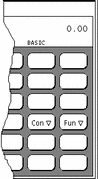Each of these keys has a menu associated with it, which you display by pressing MENU on the key. Choosing the first item from the menu displays a window that lets you enter the value of a constant or a function, and associate a name with that value. The numbers you enter are stored in a .calctoolrc file in your home directory.

Once you have entered the new value, the new value and its name are displayed in the pop-up menu associated with that Calculator key. To use the constant or function value, choose the desired item from the pop-up menu.

# Performing Simple Calculations

Calculations are performed from left to right, with no arithmetic precedence. For example, 2+3*4=20. You need parentheses, available on the Calculator base window, to establish correct arithmetic precedence. For example, 2+(3*4)=14.

To perform a numerical operation, such as adding two numbers together:

1. Click SELECT on the first digit, for example, 7.

The number is entered in the display at the upper right corner of the Calculator. In Figure 8-1 the number 0.00 is displayed.

2. Click SELECT on the operation you want to perform, for example, +.

3. Click SELECT on the number you want to add to the first number, for example, 6.

The number is displayed in the numeric display area.

4. Click SELECT on the = sign.

The result, 13 in this example, is displayed in the numeric display area.

Alternatively, you can use the keyboard to enter numbers and simple functions. You can also use the Copy key to store the numeric display to the clipboard, and the Paste key to retrieve the contents of the clipboard. If you want to copy just a portion of the numeric display, use the SELECT and ADJUST mouse buttons to define the portion of the numeric display to copy.

If an operation needing more than one input is partially complete, the pending operation is displayed on the line beneath the current number as a reminder.

# Creating and Saving a Function

Note that when you enter a value for a new function, you must type the keyboard equivalents in the Value field. To display the keyboard equivalents for each Calculator key, click SELECT on the Keys button.

The following example describes how to create and save a function that adds together two numbers taken from memory registers, and stores the result in another memory register.

1. Choose Enter Function from the Fun key menu.

The Enter Function pop-up window is displayed.

2. Type in the number of the function you wish to add or replace in the Function No: field.

The function number must be in the range 0 through 9.

3. Type a function name in the Description field, for example, addition.

4. Type a function into the Value field.

To add the numbers in Memory Registers 1 and 2, and store the sum in Register 5, type R1+R2=S5. R1 means "R[etrieve] register 1," R2 means "R[etrieve register] 2," and S5 means "S[tore] into register 5." See Table 8-1 at the end of this chapter for the keyboard equivalents (such as R and S) that you can use when creating your functions.

When you create your own functions, if you want to use a function from one of the function windows (that is, the Financial, Logical, or Scientific windows), make sure to include a command to change modes. For example, if you want to retrieve the contents of memory register 3 and get the factorial value of it, R3! will not work, because you need to change to Scientific mode before retrieving the factorial. Instead, use R3Ms!Mb. This function translates correctly to "Retrieve the contents of memory register 3, change mode to Scientific mode, get the factorial value, and change mode back to Basic mode."

5. Click SELECT on the Enter Function button to store the new function in your .calctoolrc file and add it to the Functions menu.

To use the new function, choose it from the Functions menu.

When creating functions, remember that calculations are performed from left to right, with no arithmetic precedence. For example, the calculation 2 + 3 * 4 as performed by the DeskSet Calculator gives a result of 20. The same equation performed with canonical arithmetic precedence would give a result of 14. This can be achieved with the Calculator by using parentheses: 2 + (3 * 4)

# Financial Functions

The Calculator has the financial functions shown in Figure 8-11. Choose Financial from the Mode key pop-up menu to display the Financial Mode window.

##### Figure 8-11 Financial Functions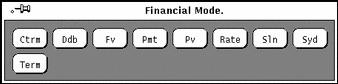The financial functions retrieve needed information from the memory registers. For example, in order to determine the amount of an installment payment, the Calculator needs to know the amount of the loan, the interest rate, and the term of the loan. You must store this information in the appropriate registers before you click SELECT on the financial function button. See "Memory Registers"to learn how to store numbers in the registers.

The following function descriptions include information about what the Calculator expects to find in each register for each function, plus examples of how to use each fu*nction.

## Ctrm: Compounding Term

Use Ctrm to compute the number of compounding periods it will take an investment of present value to grow to a future value, earning a fixed interest rate per compounding period. Store the following numbers in the memory registers:

• Register 0: Periodic interest rate

• Register 1: Future value of the investment

• Register 2: Present value of the investment

### Ctrm Example:

You have just deposited \$8,000 in an account that pays an annual interest rate of 9%, compounded monthly. You want to determine how long it will take to double you investment.

Register 0: 0.01 (interest rate = 9% / 12) Register 1: 16000 (future value) Register 2: 8000 (present value)

Clicking SELECT on Ctrm returns 92.77, which tells you that it would take 92.77 months, or almost eight years, to double your \$8,000.

## Ddb: Double-Declining Depreciation

Use Ddb to compute the depreciation allowance on an asset for a specified period of time, using the double-declining balance method. Store the following information in the memory registers:

• Register 0: Amount paid for asset

• Register 1: Salvage value of asset at end of life

• Register 2: Useful life of an asset

• Register 3: Time period for depreciation allowance

### Ddb Example:

You have purchased an office machine for \$8,000. The useful life of this machine is six years. The salvage value after six years is \$900. You want to compute the depreciate expense for the fourth year, using the double-declining balance method.

Memory register usage:

Register 0: 8000 (amount paid for asset) Register 1: 900 (value of asset at end of its life) Register 2: 6 (useful life of the asset) Register 3: 4 (time period for depreciation allowance)

Clicking SELECT on Ddb returns 790.12, which tells you that the depreciation expense for the fourth year will be \$790.12.

## Fv: Future Value

Use Fv to determine the future value of an investment. The Calculator computes the future value based on a series of equal payments, earning a periodic interest rate over the number of payment periods in a term. The memory registers need to contain the following numbers:

• Register 0: Amount of each payment

• Register 1: Interest rate

• Register 2: Number of payments

### Fv Example:

You plan to deposit \$4,000 each year for the next 20 years into a bank account. The account is paying 8% interest, compounded annually. Interest is paid on the last day of each year. You want to compute the value of your account in 20 years. You make each year's contribution on the last day of the year.

Memory register usage:

Register 0: 4000 (periodic payment) Register 1: 0.08 (periodic interest rate is 8%) Register 2: 20 (number of periods)

Clicking SELECT on Fv returns 183047.86, the value of your account in dollars at the end of 20 years.

## Pmt: Periodic Payment

Use Pmt to compute the amount of the periodic payment of a loan. Most installment loans are computed like ordinary annuities, in that payments are made at the end of each payment period. Store the following information in the memory registers:

• Register 0: Principal or amount of the loan

• Register 1: Periodic interest rate of the loan

• Register 2: Term, or number of payments

### Pmt Example:

You are considering taking out a \$120,000 mortgage for 30 years at an annual interest rate of 11.0%. You want to determine your monthly repayment.

Memory register usage:

Register 0: 120000 (principal). Register 1: 0.00916 (periodic interest rate is 11.0% / 12) Register 2: 360 (term - 30 x 12)

Clicking SELECT on Pmt returns 1142.06, the value in dollars of your monthly repayment.

## Pv: Present Value

Use Pv to determine the present value of an investment. The Calculator computes the present value based on a series of equal payments discounted at a periodic interest rate over the number of periods in the term. The following information is retrieved from the memory registers:

• Register 0: Amount of each payment

• Register 1: Periodic interest rate

• Register 2: Term, or number of payments

### Pv Example:

You have just won a million dollars. The prize is awarded in 20 annual payments of \$50,000 each (a total of \$1,000,000 over 20 years). Annual payments are received at the end of each year. You are given the option of receiving a single lump-sum payment of \$400,000 instead of the million dollars annuity. You want to find out which option is worth more in today's dollars.

If you were to accept the annual payments of \$50,000, you assume that you would invest the money at a rate of 9%, compounded annually.

Memory register usage:

Register 0: 50000 (periodic payment). Register 1: 0.09 (periodic interest rate is 9%) Register 2: 20 (term)

Clicking SELECT on Pv returns a value of 456427.28, which tells you that the \$1,000,000 paid over 20 years is worth \$456,427 in present dollars. Based on your assumptions, the lump-sum payment of \$400,000 is worth less than the million-dollar ordinary annuity, in present dollars (before taxes).

## Rate: Periodic Interest Rate

Use Rate to compute the periodic interest rate. It returns the periodic interest necessary for a present value to grow to a future value over the specified number of compounding periods in the term. Store the following information in the memory registers:

• Register 0: Future value

• Register 1: Present value

• Register 2: Term, or number of compounding periods

### Rate Example:

You have invested \$20,000 in a bond. The bond matures in five years and has a maturity value of \$30,000. Interest is compounded monthly. You want to determine the periodic interest rate for this investment.

Note -

Before entering the information into the memory registers, choose 5 radix places from the Acc (accuracy) key pop-up menu to produce more accurate results.

Memory register usage:

Register 0: 30000 (future value) Register 1: 20000 (present value) Register 2: 60 (term - 5 x 12)

Clicking SELECT on Rate returns.00678, which tells you that the periodic (monthly) interest rate is 0.678%, under 1% per month. To determine the annual rate, multiply the above formula by 12, which yields a result of 8.14%.

## Sln: Straight-line Depreciation

Use Sln to compute the straight-line depreciation of an asset for one period. The straight-line method of depreciation divides the depreciable cost (actual cost minus salvage value) evenly over the useful life of an asset. The useful life is the number of periods, typically years, over which an asset is depreciated. Use the memory registers to store the following information:

• Register 0: Cost of the asset

• Register 1: Salvage value of the asset

• Register 2: Useful life of the asset

### Sln Example:

You have purchased an office machine for \$8,000. The useful life of this machine is six years, and the salvage value in eight years will be \$900. You want to compute yearly depreciation expense, using the straight-line method.

Memory register usage:

Register 0: 8000 (cost of the asset) Register 1: 900 (salvage value of the asset) Register 2: 6 (useful life of the asset)

Clicking SELECT on Sln returns 1183.33, the yearly dollar depreciation allowance.

## Syd: Sum-of-the-years'-digits Depreciation

Use Syd to compute the sum-of-the-years'-digits depreciation. This method of depreciation accelerates the rate of depreciation so that more depreciation expense occurs in earlier periods than in later ones. The depreciable cost is the actual cost minus salvage value. The useful life is the number of periods, typically years, over which an asset is depreciated. Store the following information in the memory registers:

• Register 0: Cost of the asset

• Register 1: Salvage value of the asset

• Register 2: Useful life of the asset

• Register 3: Period for which depreciation is computed

### Syd Example:

You have just purchased an office machine for \$8,000. The useful life of this machine is six years, and the salvage value after eight years will be \$900. You want to compute the depreciation expense for the fourth year, using the sum-of-the-years'-digits method.

Memory register usage:

Register 0: 8000 (cost of the asset) Register 1: 900 (salvage value of the asset) Register 2: 6 (useful life of the asset) Register 3: 4 (period for which depreciation is computed)

Clicking SELECT on Syd returns 1014.29, the dollar depreciation allowance for the fourth year.

## Term: Payment Period

Use Term to compute the number of payment periods in the term of an ordinary annuity necessary to accumulate a future value earning a specified periodic interest rate. Store the following information in the memory registers:

• Register 0: Amount of each periodic payment

• Register 1: Future value

• Register 2: Periodic interest rate

### Term Example:

You deposit \$1,800 at the end of each year into a bank account. Your account earns 11% a year, compounded annually. You want to determine how long it will take to accumulate \$120,000.

Memory register usage:

Register 0 - 1800 (periodic payment) Register 1 - 120000 (future value) Register 2 - 0.11 (periodic interest rate is 11%)

Clicking SELECT on Term returns 20.32, the number of years it will take to accumulate \$120,000 in your account.

# Logical Functions

The Calculator has the logical functions shown in Figure 8-12. This pop-up window is displayed when you choose Logical from the Mode key pop-up menu.

##### Figure 8-12 Logical Functions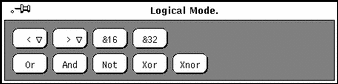• Use < to shift the displayed value, treated as a 32 bit integer, to the left. This key has a pop-up menu. The number of places to shift can be selected from this menu, and ranges from 1 through 15.

• Use > to shift the displayed value, treated as a 32 bit integer, to the right. This key has a pop-up menu. The number of places to shift can be selected from this menu, and ranges from 1 through 15.

• Use &16 to truncate the current display to a 16-bit integer.

• Use &32 to truncate the current display to a 32-bit integer.

• Use Or to perform a logical OR operation on the last number and the next number entered, treating both numbers as 32-bit integers.

• Use And to perform a logical AND operation on the last number and the next number entered, treating both numbers as 32-bit integers.

• Use Not to perform a logical NOT operation on the currently displayed value.

• Use Xor to perform a logical XOR operation on the last number and the next number entered, treating both numbers as 32-bit integers.

• Use Xnor to perform a logical XNOR operation on the last number and the next number entered, treating both numbers as 32-bit integers.

# Scientific Functions

The Calculator has the scientific functions shown in Figure 8-13. This window is displayed when you choose Scientific from the Mode key pop-up menu.

##### Figure 8-13 Scientific Functions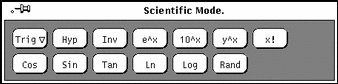• Press MENU pm the Trig key to access a pop-up menu to set the trigonometric base to Degrees, Radians, or Gradients. The current trigonometric base is indicated by the second item on the mode line.

• Use Hyp as a toggle for setting or unsetting the hyperbolic function flag. This flag affects SIN, COS, and TAN functions. When this flag is set, the word HYP appears on the mode line beneath the numeric display.

• Use Inv as a toggle for setting or unsetting the inverse function flag. This flag affects SIN, COS, and TAN trigonometric functions. When this flag is set, the word INV appears on the mode line beneath the numeric display.

• Use e^x to return e raised to the power of the currently displayed value.

• Use 10^x to return 10 raised to the power of the currently displayed value.

• Use y^x to raise the last number entered to the power of the next number.

• Use x! to return the factorial of the currently displayed value. The factorial function only works for positive integers.

• Use Cos to return the trigonometric cosine, arc cosine, hyperbolic cosine, or inverse hyperbolic cosine of the current value, depending on the settings of the hyperbolic and inverse function toggles. (See Hyp and Inv listed previously.) The result is displayed in the current trigonometric base.

• Use Sin to return the trigonometric sine, arc sine, hyperbolic sine, or inverse hyperbolic sine of the current value, depending on the settings of the hyperbolic and inverse function toggles. (See Hyp and Inv.) The result is displayed in the current trigonometric base (degrees, radians, or gradients).

• Use Tan to return the trigonometric tangent, arc tangent, hyperbolic tangent, or inverse hyperbolic tangent of the current value, depending on the settings of the hyperbolic and inverse function toggles. (See Hyp and Inv.) The result is displayed in the current trigonometric base (degrees, radians, or gradients).

• Use Ln to return the natural logarithm of the currently displayed value.

• Use Log to return the base 10 logarithm of the currently displayed value.

• Use Rand to return a random number between 0.0 and 1.0.

Click SELECT on the Set Default button to make your Appearance, Base, Mode, Display, Accuracy, Contents, Functions, and Style settings the default every time you start a new Calculator.

# Calculator Function Keys

Every function in the Calculator application has a keyboard equivalent associated with it. Table 8-1 lists each Calculator function with its keyboard equivalent. See the manual page for calctool for a more complete description of each function. The manual page for calctool also lists Calculator command line options and various resources that can be set in your .Xdefaults or .desksetdefaults files.

Keyboard equivalents listed in brackets indicate a choice of options. For example, the keyboard equivalent for the Disp function is D [e, f, s]. This means that you can enter De, Df, or Ds. Similarly, the Exch function keyboard equivalent is F [0-9], which means you can enter F0, F1, F2, etc. through F9.

Table 8-1 Calculator Function Keyboard Equivalents

Function

Keyboard Equivalent

Description

Number Manipulation Operators

Int

Control-i

Integer portion of current entry

Frac

Control-f

Fractional portion of entry

Abs

Control-u

Absolute value of current entry

+/-

Change arithmetic sign

1/x

1 divided by current entry

x^2

Square of current entry

Percentage using current entry and next entry

Sqrt

Square root

Asc

Control-a char

Displays ASCII value of character typed after Control-a

Menu Operators

Base

B [b,o,d,h]

Change the base to binary [b], octal [o], decimal [d], or hexadecimal [h]

Disp

D [e,f,s]

Change display mode to engineering [e], fixed point [f], or scientific [s]

Mode

M [b,f,l,s]

Change calculator mode to default basic mode [b], or to financial [f], logical [l], or scientific [s] mode

Acc

A[0-9]

Set display accuracy to 0 through 9 significant digits

Con

# [0-9] (defaults)

Display a constant value. The constants are labeled from 0 through 9 and can be defined by entries in the .calctoolrc file. Default values are:

0 - 0.621 (kms per hour / mph)

1 - 1.41421 (square root of 2)

2 - 2.71828 (e)

3 - 3.14159 (pi)

4 - 2.54 (cms / inch)

5 - 57.2958 (degrees in radian)

6 - 1048576.0 (2 to power of 20)

7 - 0.0353 (gms / oz)

8 - 0.948 (kilojoules /thermals)

9 - 0.0610 (cubic cms /cubic in)

Fun

F[0-9]

Execute a function. There are 10 functions, labeled 0 through 9, taken from .calctoolrc.

Rcl

R[0-9]

Retrieve value in memory register. There are 10 memory registers, labeled 0 through 9

Sto

S[0-9]

Store value in memory register 0 through 9

Exch

X[0-9]

Exchange current entry with contents of memory register 0 through 9

Other Operations

Clr

Delete

Clear calculator display

Bsp

Back Space

Delete rightmost character and recalculate

(and)

(and)

Set arithmetic precedence

Exp

Turn current entry into scientific notation mantissa. Next entry becomes exponent.

Keys

Toggle labels on calculator buttons between mouse and keyboard equivalents

Mem

Display window showing 10 memory register values

Quit

q or Q

Exit Calculator

Financial Mode Operations

Ctrm

Control-t

Compounding term

Ddb

Control-d

Double-declining depreciation

Fv

Future value

Pmt

Periodic payment

Pv

Present value

Rate

Control-r

Periodic interest rate

Sln

Control-s

Straight-line depreciation

Syd

Control-y

Sum-of-the-years`-digits depreciation

Logical Mode Operations

Term

Payment period

Shift current entry left

Shift current entry right

&16

Truncate to 16-bit unsigned integer

&32

Truncate to 32 bit unsigned integer

Or

Logical OR of current and next entries

And

Logical AND of current and next entries

Not

Logical NOT of current entry

Xor

Logical XOR or current and next entries

Scientific Mode Operations

Xnor

Logical XNOR of current and next entries

Trig

T[d,g,r]

Set trigonometrical base to degrees [d], gradians [g], or radians [r]

Hyp

Toggle hyperbolic function indicator

Inv

Toggle inverse function indicator

e^x

e raised to the power of current entry

10^x

10 raised to the power of current entry

y^x

Current entry raised to power of next entry

x!

Factorial of current entry

Cos

Control-c

Cosine of current entry

Sin

Control-s

Sine of current entry

Tan

Control-t

Tangent of current entry

Ln

Natural logarithm of current entry

Log

Base 10 logarithm of current entry

Rand

Random number from 0.0 through 1.0

# Customizing Your Calculator

Use the Calculator Properties window to customize the look and layout of your Calculator. To display the Properties window, place the pointer anywhere in the Calculator application:

Press MENU, Choose Properties from the Calculator pop-up menu, and release MENU.

The Properties window is shown in Figure 8-14.

##### Figure 8-14 Calculator Properties Window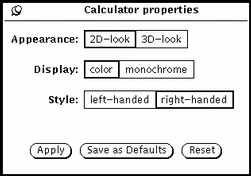• Use the Appearance setting to determine if your Calculator is displayed with two- or three-dimensional buttons. The default is two-dimensional for performance reasons.

• Use the Display setting to determine if your Calculator is shown in black-and-white or color. Color is applicable only on machines that support it.

• Use the Style setting to determine the layout of the Calculator. The default layout is with the number keys on the left. If you choose the right-handed Style setting and click SELECT on Apply or Save as Defaults, the Calculator will be laid out with the number keys on the right, as shown in Figure 8-15.

##### Figure 8-15 Right-hand Layout of Calculator Display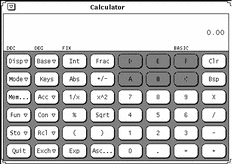Click SELECT on the Save as Defaults button to make your Appearance, Base, Mode, Display, Accuracy, Contents, Functions, and Style settings the default every time you start a new Calculator.